# Condensed Version¶

Note

Following documentation was generated automatically using a script contributed in our Discord chat. Decoded text representation may contain inaccuracies and will be revised with help from our participants.

## #1. Numbers¶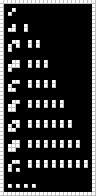0
1
2
3
4
5
6
7
8
...


## #2. Numbers (cont.)¶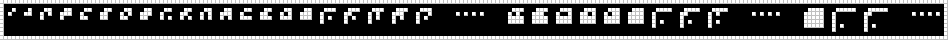Left vertical and top horizontal bars define glyph size. Number glyphs are always square. All other pixels except these bars are bits of encoded number written left to right and top to bottom. Top left pixel is always black in numbers.

0 1 2 3 4 5 6 7 8 9 10 11 12 13 14 15 16 17 18 19 20
...
506 507 508 509 510 511 512 513 514
...
65535 65536 65537
...


## #3. Negative Numbers¶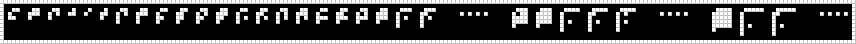Additional pixel in the vertical bar denotes a negative number.

4 3 2 1 0 -1 -2 -3 -4 -5 -6 -7 -8 -9 -10 -11 -12 -13 -14 -15 -16 -17
...
-510 -511 -512 -513 -514
...
-65535 -65536 -65537
...


## #4. Equality¶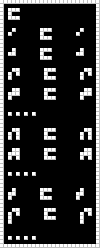=
0   =   0
1   =   1
2   =   2
3   =   3
...
10   =   10
11   =   11
...
-1   =   -1
-2   =   -2
...


## #5. Successor¶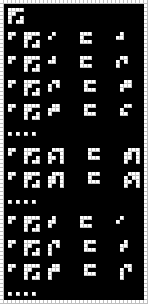inc
ap inc 0   =   1
ap inc 1   =   2
ap inc 2   =   3
ap inc 3   =   4
...
ap inc 300   =   301
ap inc 301   =   302
...
ap inc -1   =   0
ap inc -2   =   -1
ap inc -3   =   -2
...


## #6. Predecessor¶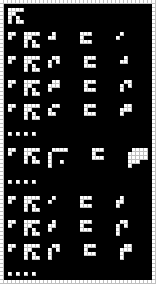dec
ap dec 1   =   0
ap dec 2   =   1
ap dec 3   =   2
ap dec 4   =   3
...
ap dec 1024   =   1023
...
ap dec 0   =   -1
ap dec -1   =   -2
ap dec -2   =   -3
...


## #7. Sum¶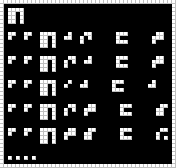add
ap ap add 1 2   =   3
ap ap add 2 1   =   3
ap ap add 0 1   =   1
ap ap add 2 3   =   5
ap ap add 3 5   =   8
...


## #8. Variables¶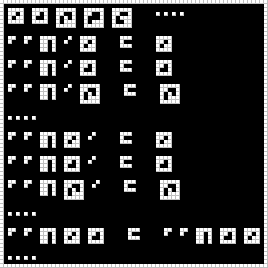Variables have a white border. Inverted number inside the border identifies the variable.

x0 x1 x2 x3 x4   ...
ap ap add 0 x0   =   x0
ap ap add 0 x1   =   x1
ap ap add 0 x2   =   x2
...
ap ap add x0 0   =   x0
ap ap add x1 0   =   x1
ap ap add x2 0   =   x2
...
...


## #9. Product¶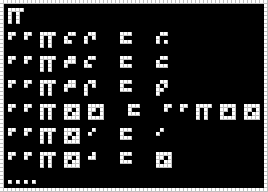mul
ap ap mul 4 2   =   8
ap ap mul 3 4   =   12
ap ap mul 3 -2   =   -6
ap ap mul x0 x1   =   ap ap mul x1 x0
ap ap mul x0 0   =   0
ap ap mul x0 1   =   x0
...


## #10. Integer Division¶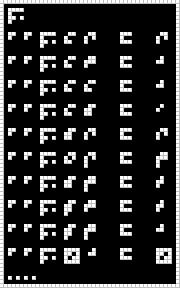Rounds toward zero.

div
ap ap div 4 2   =   2
ap ap div 4 3   =   1
ap ap div 4 4   =   1
ap ap div 4 5   =   0
ap ap div 5 2   =   2
ap ap div 6 -2   =   -3
ap ap div 5 -3   =   -1
ap ap div -5 3   =   -1
ap ap div -5 -3   =   1
ap ap div x0 1   =   x0
...


## #11. Equality and Booleans¶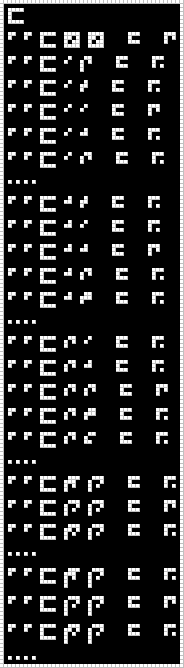t is true, f is false.

eq
ap ap eq x0 x0   =   t
ap ap eq 0 -2   =   f
ap ap eq 0 -1   =   f
ap ap eq 0 0   =   t
ap ap eq 0 1   =   f
ap ap eq 0 2   =   f
...
ap ap eq 1 -1   =   f
ap ap eq 1 0   =   f
ap ap eq 1 1   =   t
ap ap eq 1 2   =   f
ap ap eq 1 3   =   f
...
ap ap eq 2 0   =   f
ap ap eq 2 1   =   f
ap ap eq 2 2   =   t
ap ap eq 2 3   =   f
ap ap eq 2 4   =   f
...
ap ap eq 19 20   =   f
ap ap eq 20 20   =   t
ap ap eq 21 20   =   f
...
ap ap eq -19 -20   =   f
ap ap eq -20 -20   =   t
ap ap eq -21 -20   =   f
...


## #12. Strict Less-Than¶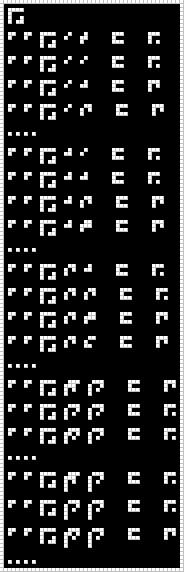lt
ap ap lt 0 -1   =   f
ap ap lt 0 0   =   f
ap ap lt 0 1   =   t
ap ap lt 0 2   =   t
...
ap ap lt 1 0   =   f
ap ap lt 1 1   =   f
ap ap lt 1 2   =   t
ap ap lt 1 3   =   t
...
ap ap lt 2 1   =   f
ap ap lt 2 2   =   f
ap ap lt 2 3   =   t
ap ap lt 2 4   =   t
...
ap ap lt 19 20   =   t
ap ap lt 20 20   =   f
ap ap lt 21 20   =   f
...
ap ap lt -19 -20   =   f
ap ap lt -20 -20   =   f
ap ap lt -21 -20   =   t
...


## #13. Modulate¶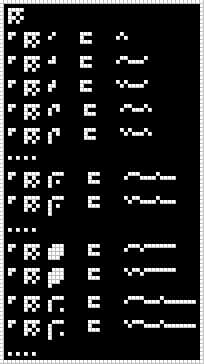mod
ap mod 0   =   
ap mod 1   =   
ap mod -1   =   [-1]
ap mod 2   =   
ap mod -2   =   [-2]
...
ap mod 16   =   
ap mod -16   =   [-16]
...
ap mod 255   =   
ap mod -255   =   [-255]
ap mod 256   =   
ap mod -256   =   [-256]
...


## #14. Demodulate¶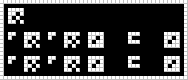dem
ap dem ap mod x0   =   x0
ap mod ap dem x0   =   x0


## #15. Send¶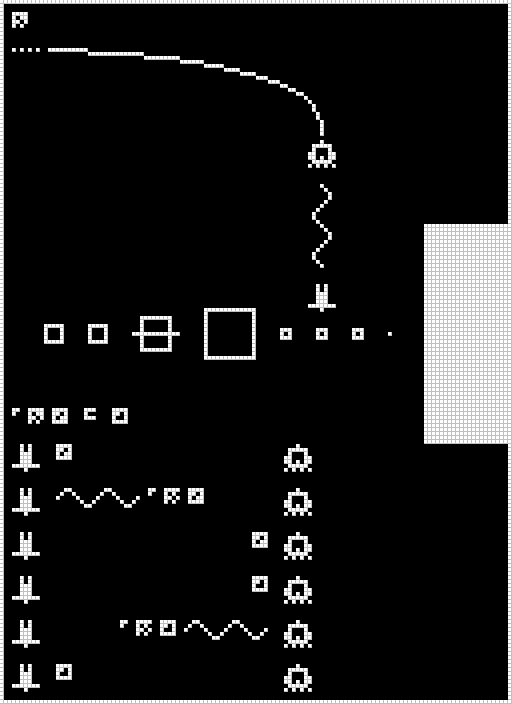send

ap send x0 =  x1

humans   x0                     aliens
humans   ~~~~~ ap mod x0        aliens
humans                       x0 aliens
humans                       x1 aliens
humans          ap mod x1 ~~~~~ aliens
humans x1                       aliens


## #16. Negate¶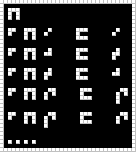neg
ap neg 0   =   0
ap neg 1   =   -1
ap neg -1   =   1
ap neg 2   =   -2
ap neg -2   =   2
...


## #17. Function Application¶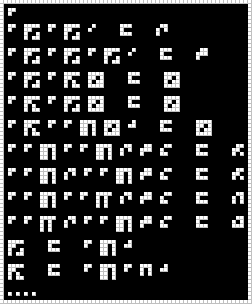ap f x is f(x)

The last two lines demonstrate that function application ap allows curried (i.e. partially evaluated) functions, by defining inc as the function x -> 1 + x.

ap
ap inc ap inc 0   =   2
ap inc ap inc ap inc 0   =   3
ap inc ap dec x0   =   x0
ap dec ap inc x0   =   x0
ap dec ap ap add x0 1   =   x0
ap ap add ap ap mul 2 3 4   =   10
ap ap mul 2 ap ap add 3 4   =   14
dec   =   ap add ap neg 1
...


## #18. S Combinator¶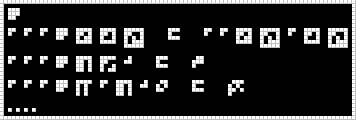s
ap ap ap s x0 x1 x2   =   ap ap x0 x2 ap x1 x2
ap ap ap s add inc 1   =   3
ap ap ap s mul ap add 1 6   =   42
...


## #19. C Combinator¶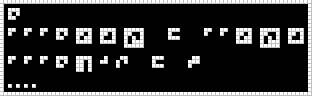c
ap ap ap c x0 x1 x2   =   ap ap x0 x2 x1
ap ap ap c add 1 2   =   3
...


## #20. B Combinator¶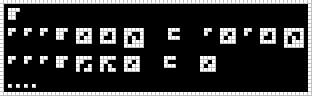b
ap ap ap b x0 x1 x2   =   ap x0 ap x1 x2
ap ap ap b inc dec x0   =   x0
...


## #21. True (K Combinator)¶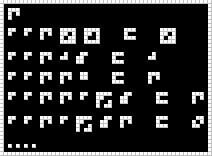Decoded as t, because it has a meaning of boolean True.

t
ap ap t x0 x1   =   x0
ap ap t 1 5   =   1
ap ap t t i   =   t
ap ap t t ap inc 5   =   t
ap ap t ap inc 5 t   =   6
...


## #22. False¶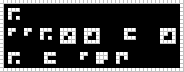Decoded as f, because it has a meaning of boolean False.

f
ap ap f x0 x1   =   x1
f   =   ap s t


## #23. Power of Two¶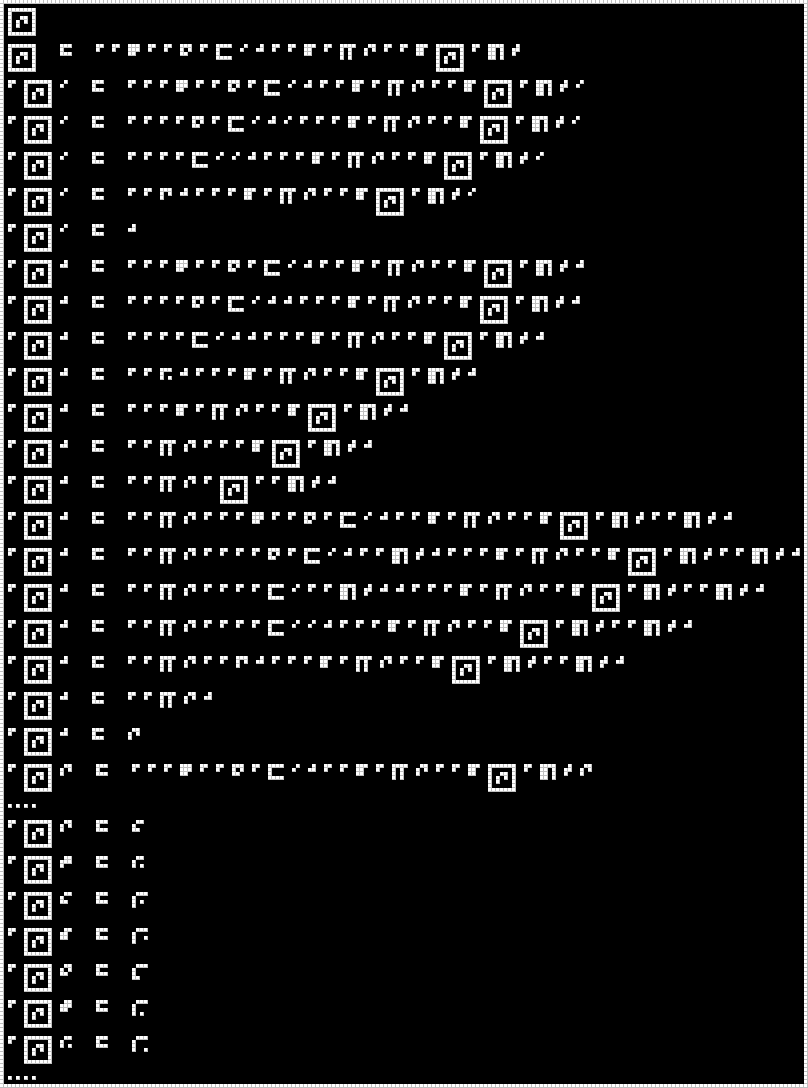Recursive function: pwr2 definition uses pwr2.

pwr2
pwr2   =   ap ap s ap ap c ap eq 0 1 ap ap b ap mul 2 ap ap b pwr2 ap add -1
ap pwr2 0   =   ap ap ap s ap ap c ap eq 0 1 ap ap b ap mul 2 ap ap b pwr2 ap add -1 0
ap pwr2 0   =   ap ap ap ap c ap eq 0 1 0 ap ap ap b ap mul 2 ap ap b pwr2 ap add -1 0
ap pwr2 0   =   ap ap ap ap eq 0 0 1 ap ap ap b ap mul 2 ap ap b pwr2 ap add -1 0
ap pwr2 0   =   ap ap t 1 ap ap ap b ap mul 2 ap ap b pwr2 ap add -1 0
ap pwr2 0   =   1
ap pwr2 1   =   ap ap ap s ap ap c ap eq 0 1 ap ap b ap mul 2 ap ap b pwr2 ap add -1 1
ap pwr2 1   =   ap ap ap ap c ap eq 0 1 1 ap ap ap b ap mul 2 ap ap b pwr2 ap add -1 1
ap pwr2 1   =   ap ap ap ap eq 0 1 1 ap ap ap b ap mul 2 ap ap b pwr2 ap add -1 1
ap pwr2 1   =   ap ap f 1 ap ap ap b ap mul 2 ap ap b pwr2 ap add -1 1
ap pwr2 1   =   ap ap ap b ap mul 2 ap ap b pwr2 ap add -1 1
ap pwr2 1   =   ap ap mul 2 ap ap ap b pwr2 ap add -1 1
ap pwr2 1   =   ap ap mul 2 ap pwr2 ap ap add -1 1
ap pwr2 1   =   ap ap mul 2 ap ap ap s ap ap c ap eq 0 1 ap ap b ap mul 2 ap ap b pwr2 ap add -1 ap ap add -1 1
ap pwr2 1   =   ap ap mul 2 ap ap ap ap c ap eq 0 1 ap ap add -1 1 ap ap ap b ap mul 2 ap ap b pwr2 ap add -1 ap ap add -1 1
ap pwr2 1   =   ap ap mul 2 ap ap ap ap eq 0 ap ap add -1 1 1 ap ap ap b ap mul 2 ap ap b pwr2 ap add -1 ap ap add -1 1
ap pwr2 1   =   ap ap mul 2 ap ap ap ap eq 0 0 1 ap ap ap b ap mul 2 ap ap b pwr2 ap add -1 ap ap add -1 1
ap pwr2 1   =   ap ap mul 2 ap ap t 1 ap ap ap b ap mul 2 ap ap b pwr2 ap add -1 ap ap add -1 1
ap pwr2 1   =   ap ap mul 2 1
ap pwr2 1   =   2
ap pwr2 2   =   ap ap ap s ap ap c ap eq 0 1 ap ap b ap mul 2 ap ap b pwr2 ap add -1 2
...
ap pwr2 2   =   4
ap pwr2 3   =   8
ap pwr2 4   =   16
ap pwr2 5   =   32
ap pwr2 6   =   64
ap pwr2 7   =   128
ap pwr2 8   =   256
...


## #24. I Combinator¶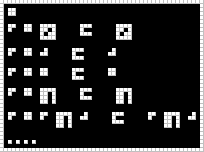i(x) = x

i
ap i x0   =   x0
ap i 1   =   1
ap i i   =   i
...


## #25. Cons (or Pair)¶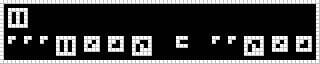cons
ap ap ap cons x0 x1 x2   =   ap ap x2 x0 x1


## #26. Car (First)¶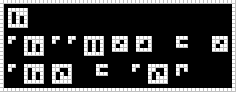car
ap car ap ap cons x0 x1   =   x0
ap car x2   =   ap x2 t


## #27. Cdr (Tail)¶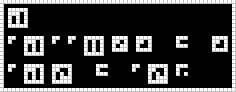cdr
ap cdr ap ap cons x0 x1   =   x1
ap cdr x2   =   ap x2 f


## #28. Nil (Empty List)¶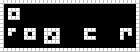nil
ap nil x0   =   t


## #29. Is Nil (Is Empty List)¶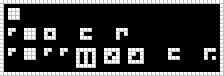isnil
ap isnil nil   =   t
ap isnil ap ap cons x0 x1   =   f


## #30. List Construction Syntax¶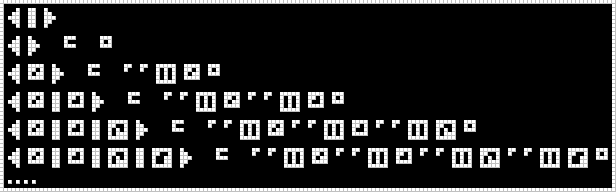( , )
( )   =   nil
( x0 )   =   ap ap cons x0 nil
( x0 , x1 )   =   ap ap cons x0 ap ap cons x1 nil
( x0 , x1 , x2 )   =   ap ap cons x0 ap ap cons x1 ap ap cons x2 nil
( x0 , x1 , x2 , x5 )   =   ap ap cons x0 ap ap cons x1 ap ap cons x2 ap ap cons x5 nil
...


## #31. Vector¶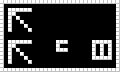Alias for cons that looks nice in “vector” usage context.

vec
vec   =   cons


## #32. Draw¶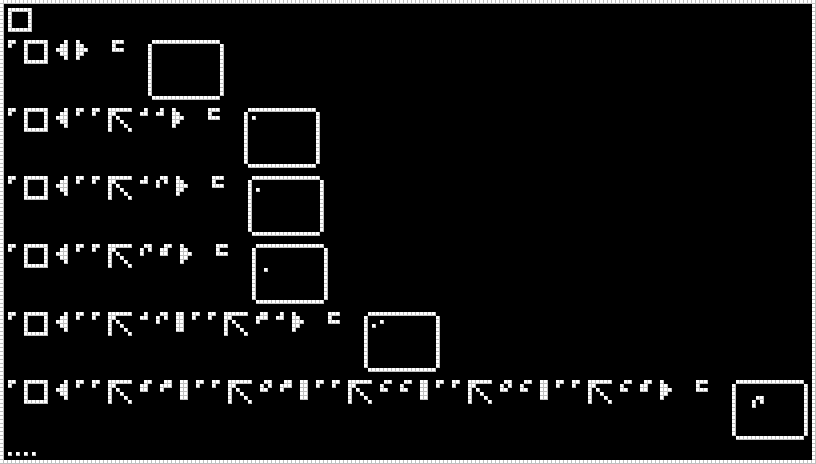It draws a list of coordinates as dots on a picture.

draw
ap draw ( )   =   |picture1|
ap draw ( ap ap vec 1 1 )   =   |picture2|
ap draw ( ap ap vec 1 2 )   =   |picture3|
ap draw ( ap ap vec 2 5 )   =   |picture4|
ap draw ( ap ap vec 1 2 , ap ap vec 3 1 )   =   |picture5|
ap draw ( ap ap vec 5 3 , ap ap vec 6 3 , ap ap vec 4 4 , ap ap vec 6 4 , ap ap vec 4 5 )   =   |picture6|
...


## #33. Checkerboard¶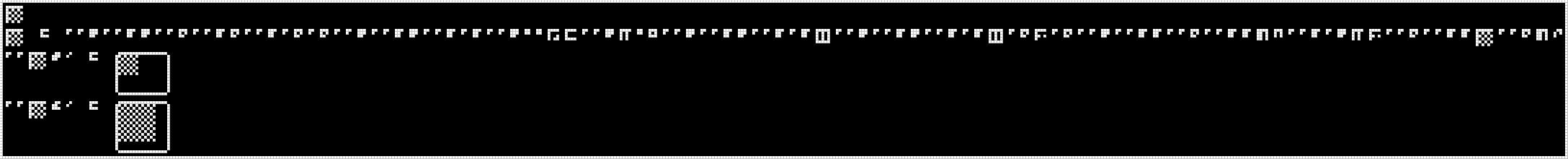Draws a checkerboard of the specified size.

checkerboard
checkerboard = ap ap s ap ap b s ap ap c ap ap b c ap ap b ap c ap c ap ap s ap ap b s ap ap b ap b ap ap s i i lt eq ap ap s mul i nil ap ap s ap ap b s ap ap b ap b cons ap ap s ap ap b s ap ap b ap b cons ap c div ap c ap ap s ap ap b b ap ap c ap ap b b add neg ap ap b ap s mul div ap ap c ap ap b b checkerboard ap ap c add 2
ap ap checkerboard 7 0   =   |picture1|
ap ap checkerboard 13 0   =   |picture2|


## #34. Multiple Draw¶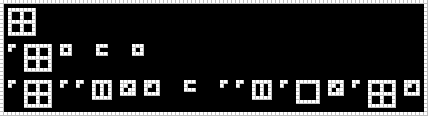Takes a list of lists of 2D-points and returns a list of rendered pictures.

It applies draw function to all items of the list.

multipledraw
ap multipledraw nil   =   nil
ap multipledraw ap ap cons x0 x1   =   ap ap cons ap draw x0 ap multipledraw x1


## #35. Modulate List¶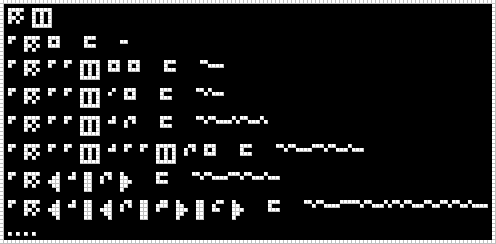Apply #13. Modulate to a list constructed with #25. Cons (or Pair) or #30. List Construction Syntax.

mod cons
ap mod nil   =   [nil]
ap mod ap ap cons nil nil   =   [ap ap cons nil nil]
ap mod ap ap cons 0 nil   =   [ap ap cons 0 nil]
ap mod ap ap cons 1 2   =   [ap ap cons 1 2]
ap mod ap ap cons 1 ap ap cons 2 nil   =   [ap ap cons 1 ap ap cons 2 nil]
ap mod ( 1 , 2 )   =   [( 1 , 2 )]
ap mod ( 1 , ( 2 , 3 ) , 4 )   =   [( 1 , ( 2 , 3 ) , 4 )]
...


## #36. Send ( 0 )¶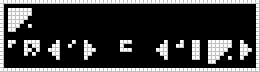:1678847 is decreasing over time at a rate of 1/3 per second and will reach 0 at the icfp contest main round deadline.

:1678847
ap send ( 0 )   =   ( 1 , :1678847 )


## #37. Is 0¶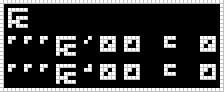Function if0 compares the first argument to 0 and picks the second argument if equal, else third.

if0
ap ap ap if0 0 x0 x1   =   x0
ap ap ap if0 1 x0 x1   =   x1


## #38. Interact¶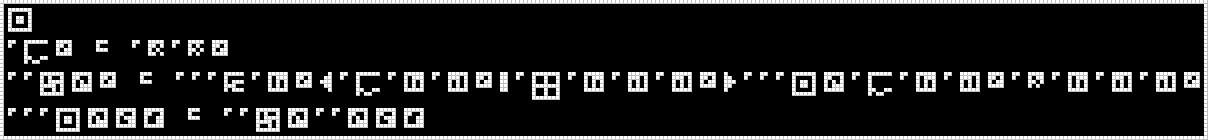Is a function that takes an “interaction-protocol”, some data (maybe “protocol” dependent), and some pixel. It returns some new data, and a list of pictures.

Note that during the execution it sometimes uses the send function to communicate with spacecraft.

// list function call notation
f38 protocol (flag, newState, data) = if flag == 0
then (modem newState, multipledraw data)
else interact protocol (modem newState) (send data)
interact protocol state vector = f38 protocol (protocol state vector)

// mathematical function call notation
f38(protocol, (flag, newState, data)) = if flag == 0
then (modem(newState), multipledraw(data))
else interact(protocol, modem(newState), send(data))
interact(protocol, state, vector) = f38(protocol, protocol(state, vector))


mod is defined on cons, nil and numbers only. So modem function seems to be the way to say that it’s argument consists of numbers and lists only.

So we can assume that newState is always list of list of … of numbers.

After experimenting with the galaxy interaction protocol we have found out several good ideas:

1. We can choose any vector to pass it to the interact function. But a convenient way to input this vectors — is clicking on the image pixel from the previous interact execution result.

1. We need to draw the images passed to multipledraw somehow. A convenient way to do it — is to overlay images one over another using different colors for different images.

interact
ap modem x0 = ap dem ap mod x0
ap ap f38 x2 x0 = ap ap ap if0 ap car x0 ( ap modem ap car ap cdr x0 , ap multipledraw ap car ap cdr ap cdr x0 ) ap ap ap interact x2 ap modem ap car ap cdr x0 ap send ap car ap cdr ap cdr x0
ap ap ap interact x2 x4 x3 = ap ap f38 x2 ap ap x2 x4 x3


## #39. Interaction Protocol¶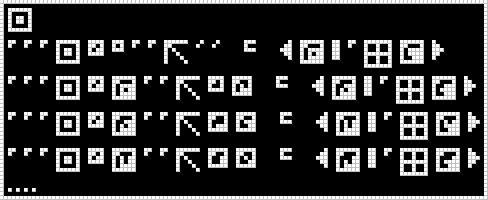Start the protocol passing nil as the initial state and (0, 0) as the initial point. Then iterate the protocol passing new points along with states obtained from the previous execution.

interact
ap ap ap interact x0 nil ap ap vec 0 0 = ( x16 , ap multipledraw x64 )
ap ap ap interact x0 x16 ap ap vec x1 x2 = ( x17 , ap multipledraw x65 )
ap ap ap interact x0 x17 ap ap vec x3 x4 = ( x18 , ap multipledraw x66 )
ap ap ap interact x0 x18 ap ap vec x5 x6 = ( x19 , ap multipledraw x67 )
...


## #40. Stateless Drawing Protocol¶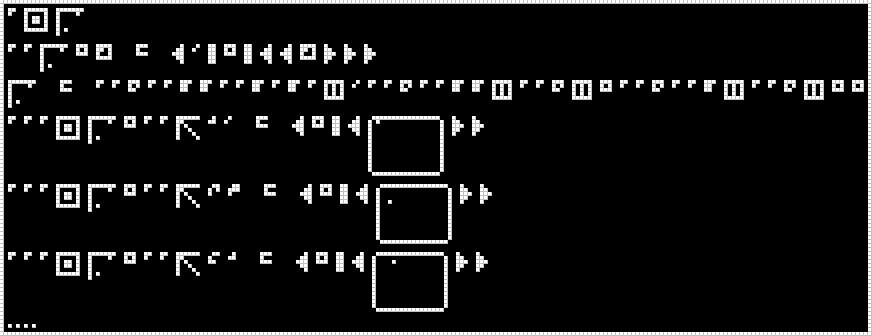ap interact statelessdraw
ap ap statelessdraw nil x1 = ( 0 , nil , ( ( x1 ) ) )
statelessdraw = ap ap c ap ap b b ap ap b ap b ap cons 0 ap ap c ap ap b b cons ap ap c cons nil ap ap c ap ap b cons ap ap c cons nil nil
ap ap ap interact statelessdraw nil ap ap vec 1 0 = ( nil , ( [1,0] ) )
ap ap ap interact statelessdraw nil ap ap vec 2 3 = ( nil , ( [2,3] ) )
ap ap ap interact statelessdraw nil ap ap vec 4 1 = ( nil , ( [4,1] ) )
...


## #41. Stateful Drawing Protocol¶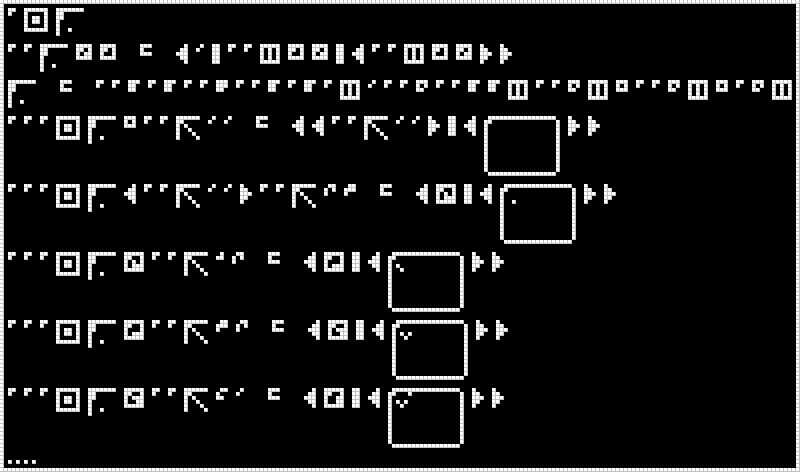It gives us back the variable bound to the draw state, so we can set the next pixel with the next call.

ap interact :67108929
ap ap :67108929 x0 x1 = ( 0 , ap ap cons x1 x0 , ( ap ap cons x1 x0 ) )
:67108929 = ap ap b ap b ap ap s ap ap b ap b ap cons 0 ap ap c ap ap b b cons ap ap c cons nil ap ap c cons nil ap c cons
ap ap ap interact :67108929 nil ap ap vec 0 0 = ( ( ap ap vec 0 0 ) , ( [0,0] ) )
ap ap ap interact :67108929 ( ap ap vec 0 0 ) ap ap vec 2 3 = ( x2 , ( [0,0;2,3] ) )
ap ap ap interact :67108929 x2 ap ap vec 1 2 = ( x3 , ( [0,0;2,3;1,2] ) )
ap ap ap interact :67108929 x3 ap ap vec 3 2 = ( x4 , ( [0,0;2,3;1,2;3,2] ) )
ap ap ap interact :67108929 x4 ap ap vec 4 0 = ( x5 , ( [0,0;2,3;1,2;3,2;4,0] ) )
...


## #42. Galaxy¶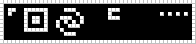We believe that this message tells us to run an interaction protocol called galaxy. This protocol is defined in the last line of the huge message included on this page.

Messages #38 and #39 describe how to run a protocol. As we can see from message #38, a protocol takes a vector and returns a list of pictures.

Messages #40 and #41 define two simple protocols and demonstrate their behavior during execution.

ap interact galaxy = ...